# 5. Union of pieces¶

In this tutorial we are going to learn to join pieces to form a single object made up of other smaller ones.

1. Open the FreeCAD application and click on the icon to create a new document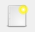.

We select the Part workbench to start designing objects in 3 dimensions.

2. At this point we are going to add the reference axes to help us place the pieces correctly.

In the menu `View... Activate or deactivate the cross of the axes.`

In English `View... Toggle axis cross`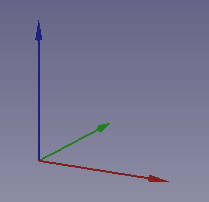3. Now we create two cubes by clicking the first icon on the solid object bar.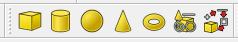We select to see the piece in isometric view.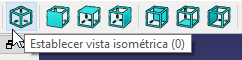The piece will look like the following image.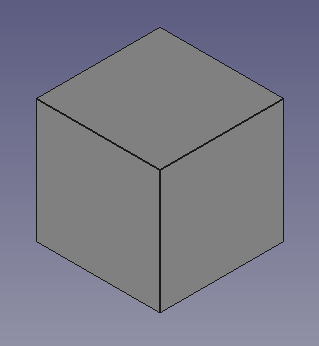4. We select the cube and in the Data tab we open the Box menu where we will see the Length, Width and Height of the cube (Length, Width, Height).

We modify the size of the two cubes to get the piece that can be seen in the following figure.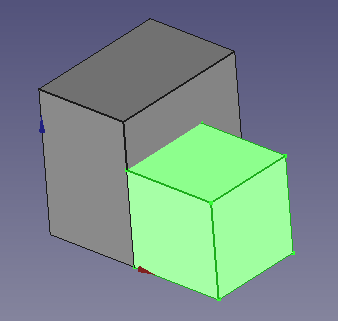The positions and sizes will be as follows.

Cube: x=0, y=0, z=0, Length=10, Width=15, Height=15

Cube 001: x=10, y=0, z=0, Length=10, Width=10, Height=10

5. Now we are going to join the two pieces created.

First we select the two cubes by clicking on them while pressing the letter Control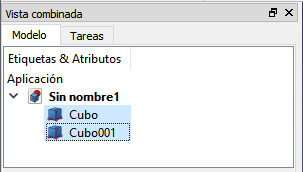Next we click the Make union of several forms icon from the Boolean toolbar.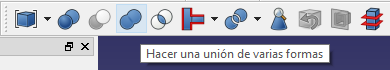Or we select from the menu `Piece... Boolean... Union`.

A new Fusion composed of the two previous pieces will be created.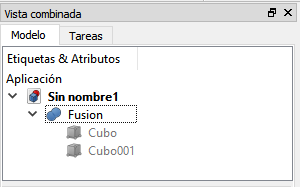6. The Fusion object will behave as a single object, so that if we change its position, all its pieces change position together.

Try changing the position of the Fusion object to see how it moves.

For example, changing the position x = 5, y = 5, z = 0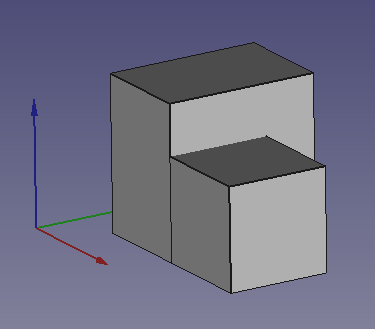7. Now we are going to simplify the faces of the object so that it looks like a single piece and not several pieces joined together.

To achieve this, we select the Fusion object In the Data tab, within Boolean, we change the Refine value to true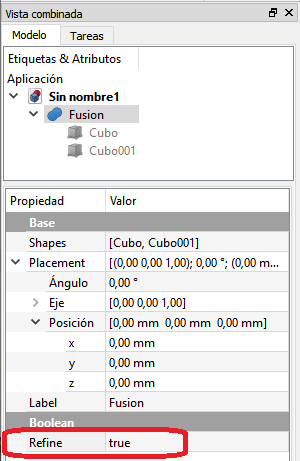With this option changed, the different parts will now have been joined into a single solid.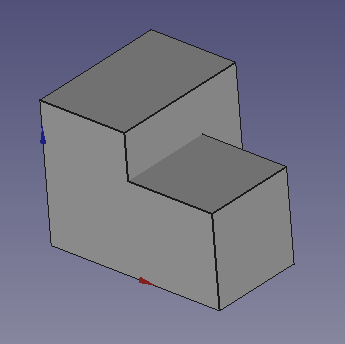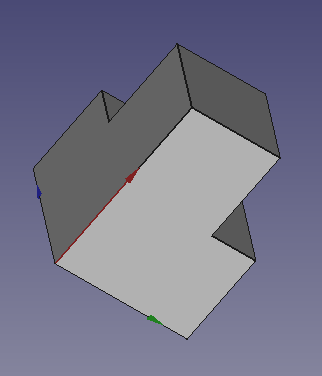Another way to get the object faces simplified is to select all the pieces and use the `Part... Refine Shape` menu.

8. If we now select Fusion and delete it (Delete key or `Edit...Delete` menu) the union of the two pieces will disappear, but the pieces will continue to exist as separate elements.

## Exercises¶

1. Create the following figure by changing the size and position of several cubes.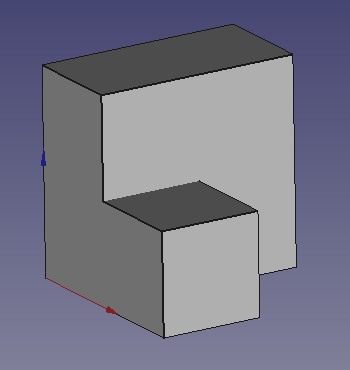The positions and sizes will be as follows.

Cube: x=0, y=0, z=0, Length=10, Width=20, Height=20

Cube 001: x=10, y=0, z=0, Length=10, Width=10, Height=10

Finally, combine the different cubes to form a single piece.

2. Create the following figure by changing the size and position of several cubes.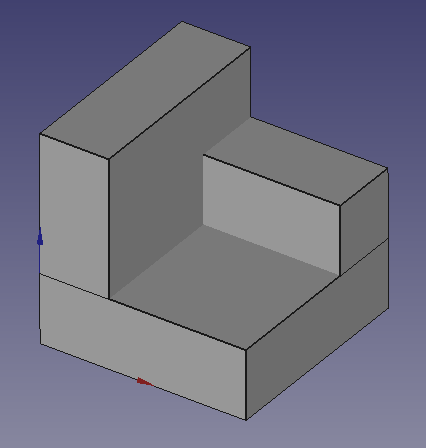Combine the different cubes to form a single piece.

3. Create the following figure by changing the size and position of several cubes.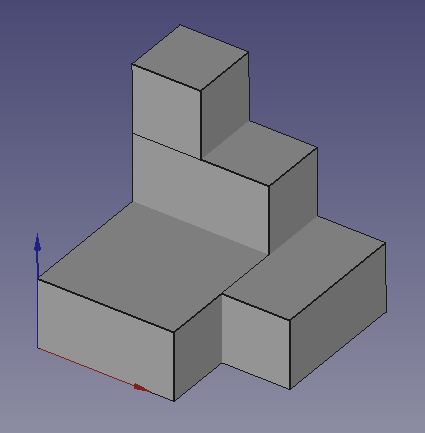Combine the different cubes to form a single piece.

4. Create the following figure by changing the size and position of several cubes.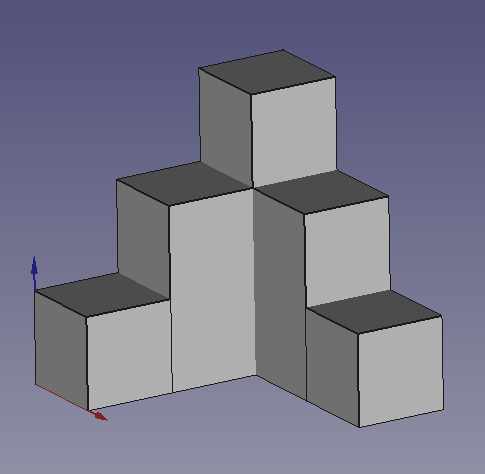Combine the different cubes to form a single piece.

5. Create the following figure by changing the size and position of several cubes.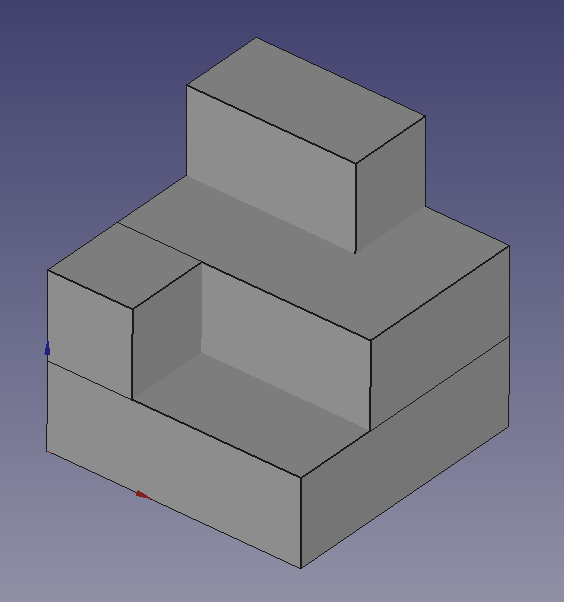Combine the different cubes to form a single piece.

6. Create the following figure by changing the size and position of several cubes.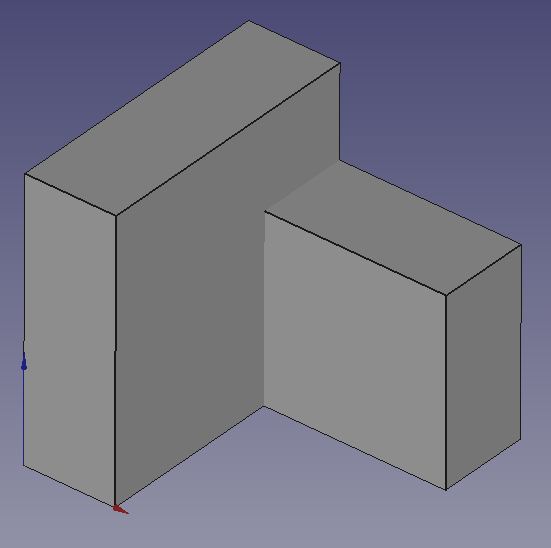Combine the different cubes to form a single piece.

## Video-tutorial¶

Union is strength.